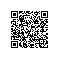# CSS入门经典-知识点摘录

Width属性的“收缩”和“扩展”适应

1、 设一个变量a,若样式是通过(X)HTMLstyle属性应用的，a的值为1，否则为0.

2、 计算选择器中ID属性的数量，和为变量b的值。

3、 计算选择器中属性、伪类和类名的数量，三者之和为变量 c的值。

4、 计算选择器中元素名的数量，和为变量d的值。

5、 忽略伪元素

.把每个变量中的数字连接在一起得到选择器的针对性。连接是一个编程术语，表示粘合。在这里，将abcd连接得到abcd，而不是计算abcd之和得到另一个变量e

 选择器 选择器类型 针对性 * 通用选择器 0000 (a=0,b=0,c=0,d=0) li 元素名 0001 (a=0,b=0,c=0,d=1) ul li 元素名 0002 (a=0,b=0,c=0,d=2) div h1+p 元素名 0003 (a=0,b=0,c=0,d=3) input[type=’text’] 元素名+属性 0011 (a=0,b=0,c=1,d=1) .someclass 类名 0010 (a=0,b=0,c=1,d=0) div.someclass 元素名+类名 0011 (a=0,b=0,c=1,d=1) div.someclass.someother 元素名+类名+类名 0021 (a=0,b=0,c=2,d=1) #someid ID名 0100 (a=0,b=1,c=0,d=0) div#someid 元素名+ID名 0101 (a=0,b=1,c=0,d=1) style(属性) style(属性) 1000 (a=1,b=0,c=0,d=0)

important规则

P{

Background:lightblue !important;

}

margin属性来水平对齐元素

atuo关键字和margin属性一起协作可以左对齐、居中对齐或者右对齐一个元素。

IE(有别于标准)可以使用text-align属性来对齐元素。这个技术在其他浏览器中不起作用。

1、 浮动元素的外边距不会折叠，不管它们如何靠近

2、 只有浮动元素后面的元素内容会被该浮动元素所影响。就是说浮动元素后面元素的背景、外边框、边框、内边距和宽度(盒模型和尺寸)都不受影响。

3、 浮动元素总是会被当作块级元素来对待

1、 相对定位类似于静态定位，其元素保持在正财的文档流中，但这也是仅有的相似之处。

2、 相对定位元素可以用作绝对定位元素的参考点。

3、 相对定位元素可以接受4种偏移属性的组合方式有：toplefttoprightbottomleftbottomright，浏览器将忽略除此之外的其他种组合方式。例如，不能对一个相对定位的元素同时使用topbottom偏移属性。

4、 相对定位的内容可以堆叠和分层放置在Z轴上使用钉钉扫一扫加入圈子
+ 订阅